# Triangle - math word problems

1. Concentric circlesIn the circle with diameter 19 cm is constructed chord 9 cm long. Calculate the radius of a concentric circle that touches this chord.
2. Triangular pyramidIt is given perpendicular regular triangular pyramid: base side a = 5 cm, height v = 8 cm, volume V = 28.8 cm3. What is it content (surface area)?
3. Again saw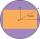From the trunk of the tree we have to a sculpture beam with rectangular cross-section with dimensions 146 mm and 128 mm. What is the trunk smallest diameter?
4. BevelI have bevel in the ratio 1:6. What is the angle and how do I calculate it?
5. Angles and sides of the triangle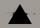Triangle ABC has a circumference of 26 cm. Lengths of the sides are as follows: a = 11.2 cm; b = 6.5 cm. Arrange the interior angles in order of its size. ?
6. MedianIn triangle ABC is given side a=10 cm and median ta= 13 cm and angle gamma 90°. Calculate length of the median tb.
7. Goat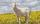Meadow is a circle with radius r = 19 m. How long must a rope to tie a goat to the pin on the perimeter of the meadow to allow goat eat half of meadow?
8. Right angled triangleHypotenuse of a right triangle is 17 cm long. When we decrease length of legs by 3 cm then decrease its hypotenuse by 4 cm. Determine the size of legs.
9. RT and ratioA right triangle whose legs are in a ratio 6:12 has hypotenuse 68 m long. How long are its legs?
10. TriangleCalculate the sides of the triangle if its area S = 630 and the second cathethus is shorter by 17.
11. Isosceles trapezoidCalculate the area of an isosceles trapezoid whose bases are in the ratio of 4:3; leg b = 13 cm and height = 12 cm.
12. Trapezoid - diagonalTrapezoid has a length of diagonal AC corssed with diagonal BD in the ratio 2:1. The triangle created by points A, cross point of diagonals S and point D has area 164 cm2. What is the area of the trapezoid?
13. Spherical cap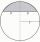What is the surface area of a spherical cap, the base diameter 20 m, height 2 m.
14. ISO triangleCalculate the area of an isosceles triangle KLM if the length of its sides are in the ratio k:l:m = 4:4:3 and has perimeter 377 mm.
15. Parallelogram ABCDThe area of parallelogram ABCD is 440 cm2. Points M and N are the midpoints of the sides AB and BC. What is the area of a quadrilateral MBND?
16. Obtuse angle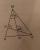The line OH is the height of the triangle DOM, line MN is the bisector of angle DMO. obtuse angle between the lines MN and OH is four times larger than the angle DMN. What size is the angle DMO? (see attached image)
17. Cathethus and the inscribed circleIn a right triangle is given one cathethus long 14 cm and the radius of the inscribed circle of 5 cm. Calculate the area of this right triangle.
18. BuildingThe building I focused at an angle 30°. When I moved 5 m building I focused at an angle 45°. What is the height of the building?
19. ISO Triangle V2Perimeter of RR triangle (isosceles) is 474 m and the base is 48 m longer than the arms. Calculate the area of this triangle.
20. TreeHow tall is the tree that observed in the visual angle of 52°? If I stand 5 m from the tree and eyes are two meters above the ground.

Do you have an interesting mathematical word problem that you can't solve it? Submit math problem, and we can try to solve it.

We will send a solution to your e-mail address. Solved examples are also published here. Please enter the e-mail correctly and check whether you don't have a full mailbox.

Please do not submit problems from current active competitions such as Mathematical Olympiad, correspondence seminars etc...